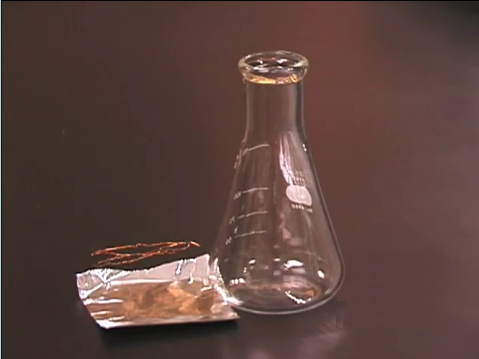Search:

# Determination of molar masses (by gas laws)

Click on the image !Here is an Erlenmeyer flask, an aluminum foil and a copper filament: the total mass is accurately determined: m1

## Principle

Mass of the vapour = $m = m_2-m_1$ Absolute temperature = $T=273,15+t$ Barometric pressure = $p$ Gas law: Number of moles = $n=\frac{pV}{RT}$ Molar mass = $M=\frac{m}{n}$

## Example

Experimental data: Mass(erlen+foil+filament) = $90.4576\; g$ Mass(erlen+foil+filament+substance) = $90.8488\; g$ Boiling point of water = $100.5\;^oC$ Volume of the Erlenmeyer flask= $154\; mL$ Atmospheric pressure = $746.5\; mmHg$ Calculation: Mass of the vapour = $m$ $=$ $90.8488-90.4576$ $=$ $0.3912\; g$ Absolute temperature = $T$ $=$ $273.15+100.5$ $=$ $373.65 \;K$ Barometric pressure = $p$ $=$ $\frac{746.5}{760}$ $=$ $0.9822\; atm$ Gas law: Number of moles = $n$ $=$ $\frac{pV}{RT}$ $=$ $\frac{0.982\cdot 0.154}{0.0821\cdot 373,65}$ $=$ $0.00492 mol$ Molar mass = $M=$ $\frac{m}{n}$ $=$ $\frac{0.3912}{0.00492}$ $=$ $79,5 \; \frac{g}{mol}$